# Determine the value of $x$, if $\overline{AB}$ divides $\angle DAC$ in ratio $1:3$ and $AB = DB$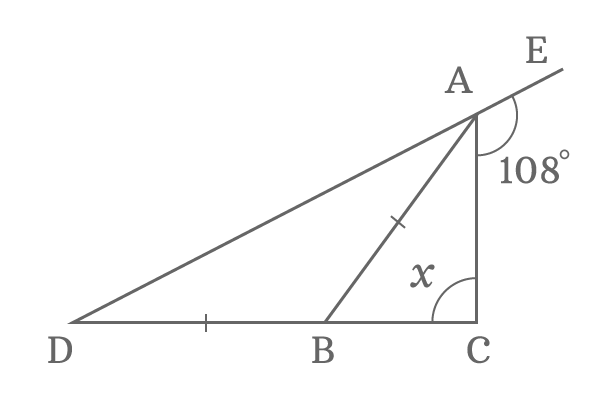$\overline{AB}$ is a common side of two triangles $BDA$ and $BCA$. It divides the angle of the triangle $DAC$ in the ratio $1:3$. The side $\overline{DA}$ of $\Delta DAC$ is extended externally to have an exterior angle $108^\circ$.

Similarly, the length of the side $\overline{AB}$ is exactly equal to the length of the side $\overline{BD}$.

It is required to determine the value of x by using the geometric data in this geometry problem.

###### Step: 1

The line segment $\overline{AB}$ divides the $\angle DAC$ in the ratio $1:3$. Geometrically, the $\angle DAB$ is less than the $\angle BAC$.

$\dfrac{\angle DAB}{\angle BAC} = \dfrac{1}{3}$

$\implies 3 \angle DAB = \angle BAC$

$\implies \angle BAC = 3 \angle DAB$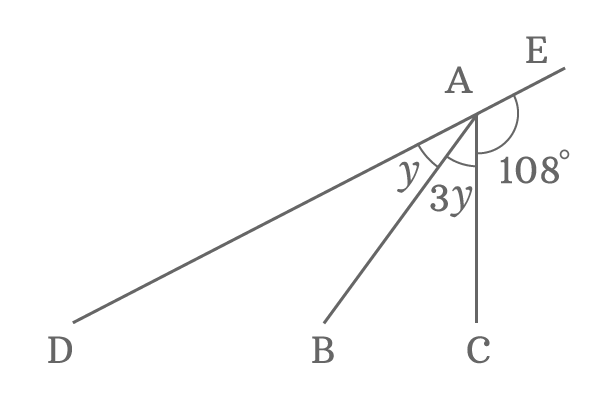Take $\angle DAB = y$. Therefore, $\angle BAC = 3y$.

The line segment $\overline{DE}$ is a straight line. So, it is angle is $\angle DAE$ and it is $180^\circ$ geometrically. The line segments $\overline{AB}$ and $\overline{AC}$ divided the straight angle into three sub angles. The sum of them is equal to the angle of the straight line.

$\angle DAB + \angle BAC + \angle CAE = 180^\circ$

$\implies y+3y+108^\circ = 180^\circ$

$\implies 4y = 180^\circ -108^\circ$

$\implies 4y = 72^\circ$

$\implies y = \dfrac{72^\circ}{4}$

$\therefore \,\,\,\,\,\, y = 18^\circ$

Therefore, $\angle DAB = y = 18^\circ$ and $\angle BAC = 3y = 3 \times 18^\circ = 54^\circ$

###### Step: 2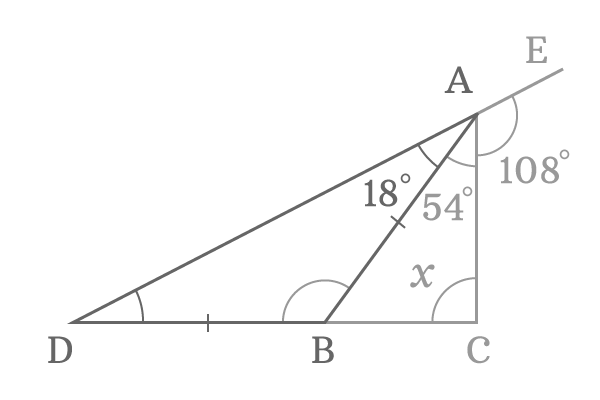$\Delta BDA$ is a triangle. One angle $\angle DAB$ is known and it is $18^\circ$ but the other two angles $\angle ABD$ and $\angle BDA$ are unknown. It is not possible to find two angles at a time.

However, it is mentioned in our problem that the lengths of sides $\overline{AB}$ and $\overline{BD}$ are equal. In other words $AB = DB$. Therefore, the $\Delta BDA$ is an isosceles triangle. According to properties of triangle, the angles opposite to equal length sides are equal.

In $\Delta BDA$, $\overline{AB}$ and $\overline{BD}$ are sides of equal length and their opposite angles are $\angle BDA$ and $\angle DAB$ and they are equal geometrically.

$\therefore \,\,\,\,\,\, \angle BDA = \angle DAB = 18^\circ$

Now, two angles are known for the triangle $BDA$ and the third angle can be determined by applying sum of the angles of a triangle property.

$\implies \angle ABD + \angle BDA + \angle DAB = 180^\circ$

$\implies \angle ABD + 18^\circ + 18^\circ = 180^\circ$

$\implies \angle ABD + 36^\circ = 180^\circ$

$\implies \angle ABD = 180^\circ –36^\circ$

$\therefore \,\,\,\,\,\, \angle ABD = 144^\circ$

###### Step: 3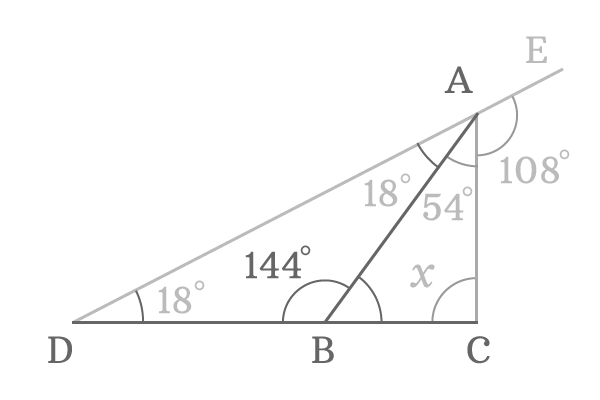$\overline{CD}$ is a straight line and its angle $CBD$ is $180^\circ$ geometrically. The $\angle CBD$ is divided by the line segment $\angle AB$. One side of the angle $DBA$ is known and it is $144^\circ$ and the other side of the angle is unknown but it can be determined by the angle $ABD$.

$\angle ABD + \angle ABC = \angle CBD$

$\implies 144^\circ + \angle ABC = 180^\circ$

$\angle ABC = 180^\circ -144^\circ$

$\therefore \,\,\,\,\,\, ABC = 36^\circ$

###### Step: 4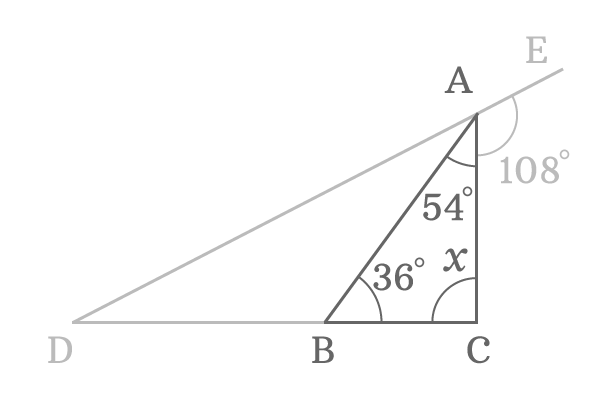The value of $x$ can be determined and it represents the angle $BCA$ in triangle $BCA$. In $\Delta BCA$, the remaining two angles are known. So, use sum of interior angles rule of a triangle to find it geometrically.

$\angle CBA + \angle BAC + \angle ACB = 180^\circ$

$\implies 36^\circ + 54^\circ + x = 180^\circ$

$\implies 90^\circ + x = 180^\circ$

$\implies x = 180^\circ -90^\circ$

$\therefore \,\,\,\,\,\, x = 90^\circ$

Latest Math Topics
Latest Math Problems

A best free mathematics education website for students, teachers and researchers.

###### Maths Topics

Learn each topic of the mathematics easily with understandable proofs and visual animation graphics.

###### Maths Problems

Learn how to solve the maths problems in different methods with understandable steps.

Learn solutions

###### Subscribe us

You can get the latest updates from us by following to our official page of Math Doubts in one of your favourite social media sites.